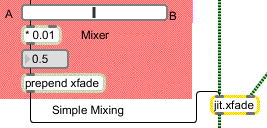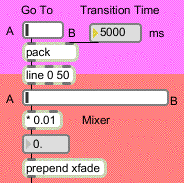Tutorial 8: Simple Mixing
Mixing Two Video Sources
One of the most common and useful things to do with video is to mix two images together.
The simplest form of video mixing is achieved by just adding the two images together, with the intensity of each of the two images adjusted in whatever proportion you desire. By fading one image up in intensity while fading the other down, you can create a smooth crossfade from one image to the other.
Jitter provides an object that accomplishes mixing and crossfading for you, called jit.xfade.
Technical Detail: This type of mixing involves adding each value in one matrix to the corresponding value in the other matrix—cell-by-cell and plane-by-plane—and outputting a matrix that contains all the sums. If we just did that, however, we would get an output image in which all the values are greater than in either of the input images, so the result would be lighter than the originals (and some of the values might even clip at 255 if the matrices contain char data). Therefore, it's normal to scale down the intensity of one or both of the images before adding them together. For example, to get an equal mix of the two images, we would scale them both down by an equal amount (say, by a factor of 0.5) before adding them.
The jit.xfade object takes a matrix in each of its two inlets, scales the values of each matrix by a certain amount, adds the two matrices together, and sends out a matrix that contains the resulting mix. The scaling factor for each of the two input matrices is determined by the object's xfade attribute. The xfade value is a single (float) number between 1 and 0. That value determines the scaling factor for the matrix coming in the right inlet. The matrix coming in the left inlet is scaled by a factor of 1-xfade. So, if you gradually increase the xfade value from 0 to 1, the output matrix will crossfade from the left input to the right input.
• Open the tutorial patch 08jSimpleMixing in the Jitter Tutorial folder. Two source videos are read in automatically by the loadbang object. Start the metro. You'll see only the left video at first. Drag on the slider to change the xfade value, which will give you a mix of the left and right matrices.A value of 0.5 gives an equal mix of left and right matrices
A crossfade is one of the most common ways of making a transition from one image to another. It might be very gradual—over the course of several seconds—or it might be quite rapid, lasting a fraction of a second, to make a sudden transition that is slightly smoother than a jump cut.
In the upper left portion of the patch, we've made an automated fader from video A to video B (or vice versa). The crossfade can take any amount of time; you can specify the transition time with the number box.Automated process to send a continuously changing xfade value
• Using the number box, set a slow transition time (say, 5000 ms) so that you can see the effect of the crossfader. Click on right side of the Go To switch to fade to video B.
The Go To switch is actually a small slider with a range of 2 and a multiplier of 100, so the only two values it can send out are 0 and 100. Clicking on the right side of the switch sends out the value 100, the pack object sends out the message 100 5000, and the line object sends out continuous values from 0 to 100 (one new value every 50 ms) over the course of five seconds. Those values are then multiplied by 0.01 to give a smoothly changing xfade value from 0 to 1.
Summary
Adding two matrices together is the simplest way to do A-B mixing of video images. To achieve the desired balance of the two images, you first scale each matrix by a certain factor. You can crossfade from one image to another by decreasing the scaling factor of one image from 1 to 0 as you increase the scaling factor of the other image from 0 to 1.
The jit.xfade object makes it easy to mix and/or crossfade two matrices. Its xfade attribute determines the balance between the two matrices. Changing the xfade value continuously from 0 to 1 performs a smooth A-B crossfade. You can use line or any other Max timing object to automate crossfades.